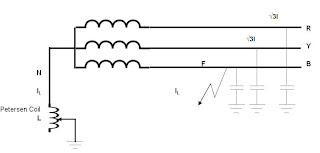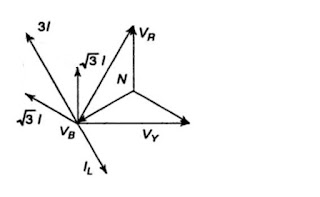Petersen Coils - Principle and Application

Peterson coils are used to in ungrounded 3-phase grounding systems to limit the arcing currents during ground faults. The coil was first developed by W. Petersen in 1916.

Application:

When a phase to earth fault occurs in ungrounded 3 phase systems, the phase voltage of the faulty phase is reduced to the ground potential. This causes the phase voltage in the other two phases to rise by √3 times. This increase in voltage causes a charging current, Ic between the phase-to-earth capacitances. The current Ic, whichincreases to three times the normal capacitive charging current, needs to complete its circuit. This causes a series of restrikes at the fault locations known as arcing grounds. This can also lead to overvoltages in the system.

A Petersen coil consists of an iron-cored reactor connected at the star point of a three phase system. In the event of a fault, the capacitive charging current is neutralized by the current across the reactor which is equal in magnitude but 180 degrees out of phase. This compensates for the leading current drawn by the line capacitances.The power factor of the fault moves closer to unity. This facilitates the easy extinguishing of the arc as both the voltage and current have a similar zero-crossing.

IC=3I=3Vp/(1/ωC) =3VpωC

Where IC is the resultant charging current that is three times the charging current of each phase to ground.

Consider a Petersen coil connected between the star-point and the ground with inductive reactance ωL, then

The current flowing through it is given by

IL =Vp/ωL

To obtain an effective cancellation of the capacitive charging currents, IL to be equal to IC.

Therefore,

Vp/ωL=3VpωC

From which we get,

L=1/ (3ω2C)

The value of the inductance in the Petersen coil needs to match the value of the line capacitance which may vary as and when modifications in the transmission lines are carried out. Hence, the Petersen coil comes with a provision to vary the inductance.